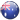# AUS:+61-280-07-5697

This law describes the volume-amount relationship of gases at constant temperature and pressure. It was given by Amedeo Avogadro in 1811. It states that equal volumes of all the gases under similar conditions of temperature and pressure contain equal number of molecules.

For example, 1 mol of all the gases contain 6.023 x 1023 molecules. At the same time 1 mol of all the gases at 273. I 5 K (0°C) and 100 kPa pressure occupy a volume of 22.7 L (22.7 x 10-3 m3 ). This means that as long as the temperature and pressure remain constant the volume of the gas is directly proportional to the number of molecules or in other words. the amount of the gas. Mathematically we can write

V – N                          (T and P are constant)

The number of molecules N is directly proportional to number of moles (n)

V= N                           (T and P are constant)

or                                 V = k3n, k3 is constant of proportionality

Now, if m is the mass of the gas having molar mass equal to M, then the number of moles (n) are given as

n = m / M

V = K4 m / M

M = K4 m / M

M  = K4 m/V or k4 d

where d is density of gas.

The above relationship implies that density of the gas at a given temperature and pressure is directly proportional to its molar mass